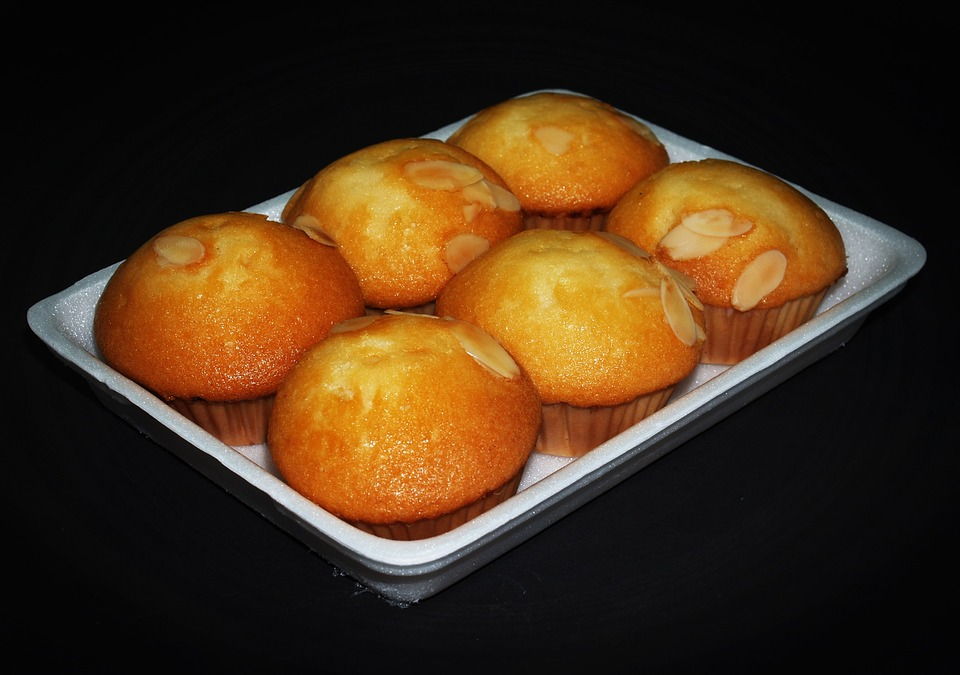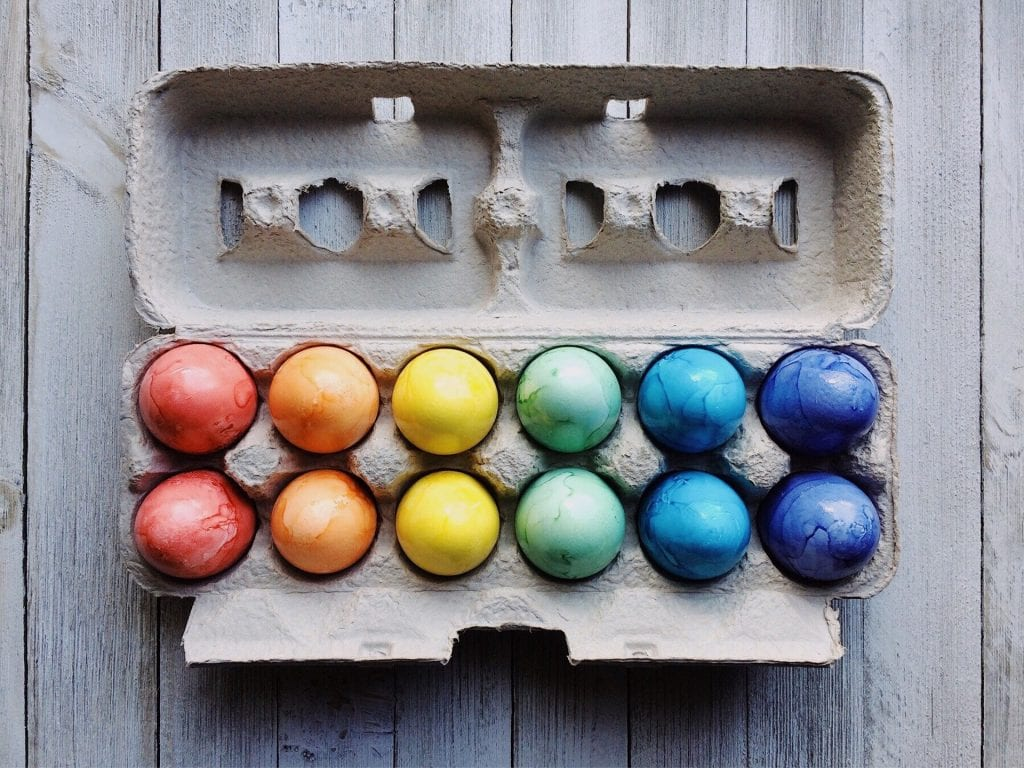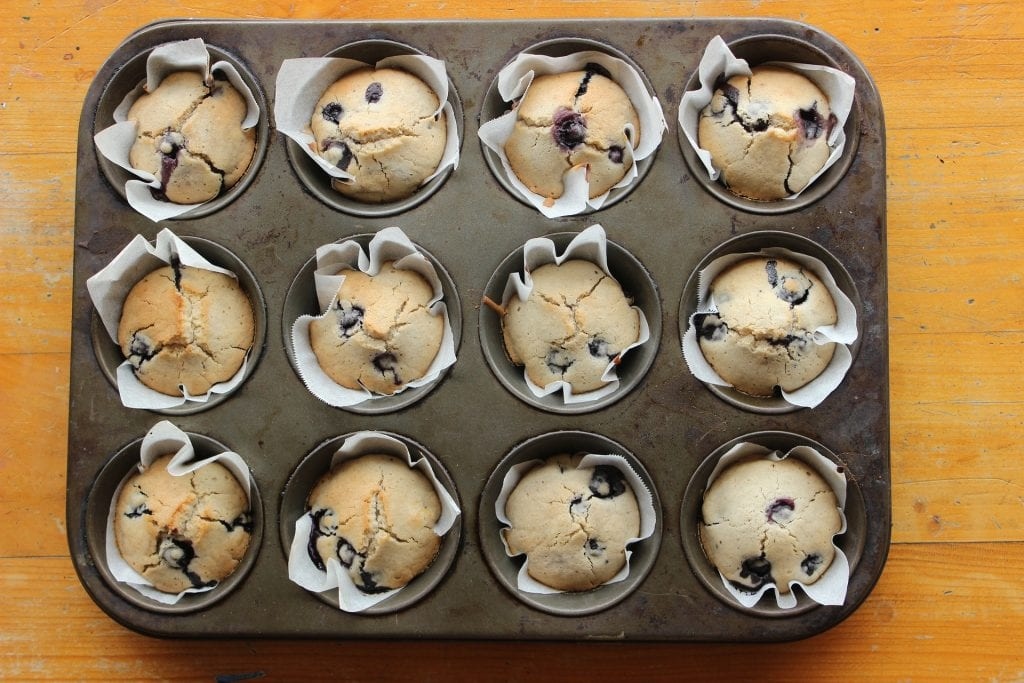# How to teach multiplication to KS1 children? Examples + FREE Printable

Mastering multiplication is such an important skill for KS1 children. Children, who don’t understand basic multiplication fully, struggle later while trying to learn more challenging math concepts. Once kids master multiplication, all other math concepts are so much easier to learn. We have explained with examples all the strategies that will help parents and teachers “How to teach multiplication?” to Year 1 and Year 2 children.

The four multiplication strategies that are introduced in KS1 are:

2. Equal groups
3. Arrays
4. Number line.

Related: Multiplication – Times Table for ages 7 to 11

Starting with something that children already know helps build confidence in children. The repeated addition strategy introduces kids to the idea of multiplication. Children find it easy to grasp as they have already mastered the concept of addition. In repeated addition, you add the same number again and again in order to find the answer to a multiplication problem.

Example: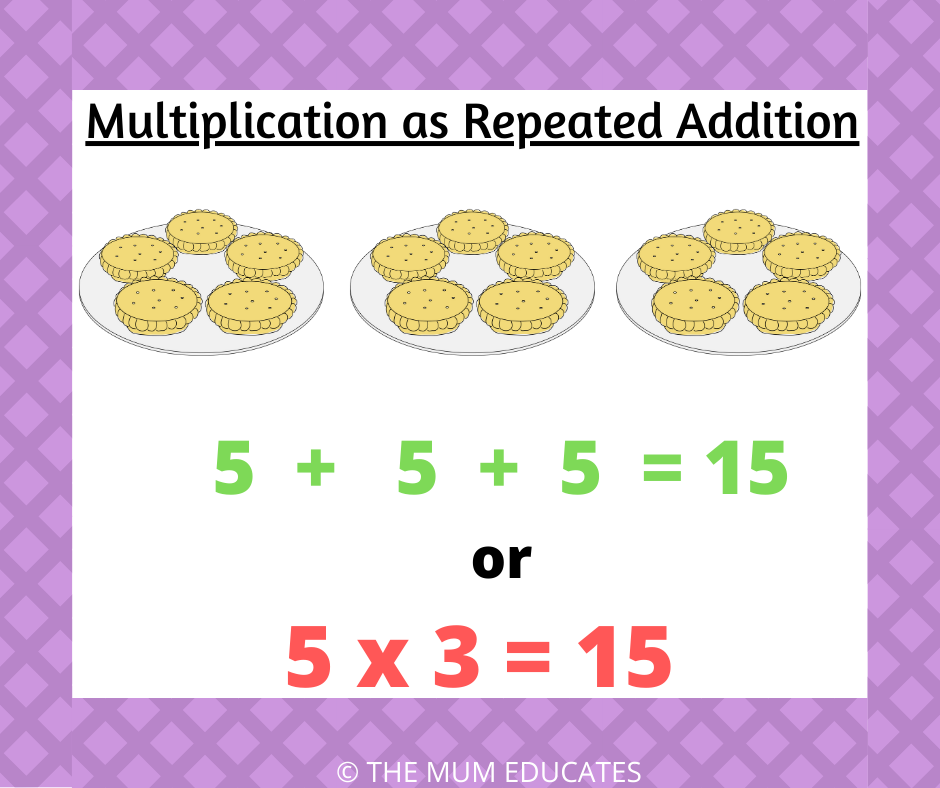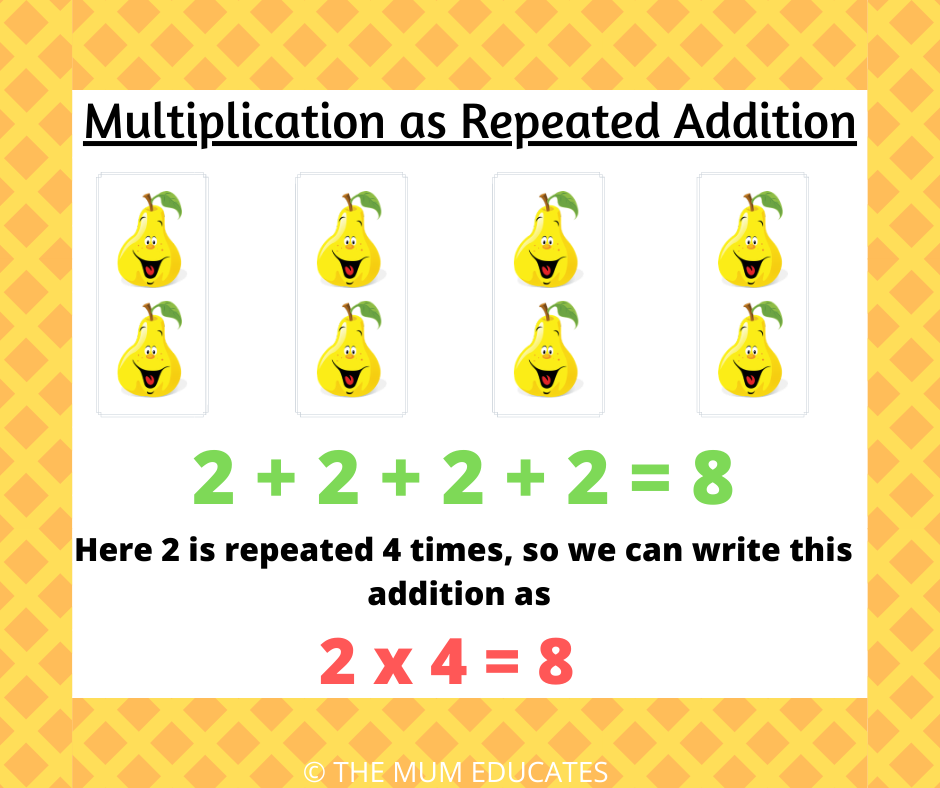2. Equal groups:

Another way to solve the multiplication problem is by making equal groups. To make Equal groups put the same number of objects in each group.

Example:

There are five groups with two flowers in each group.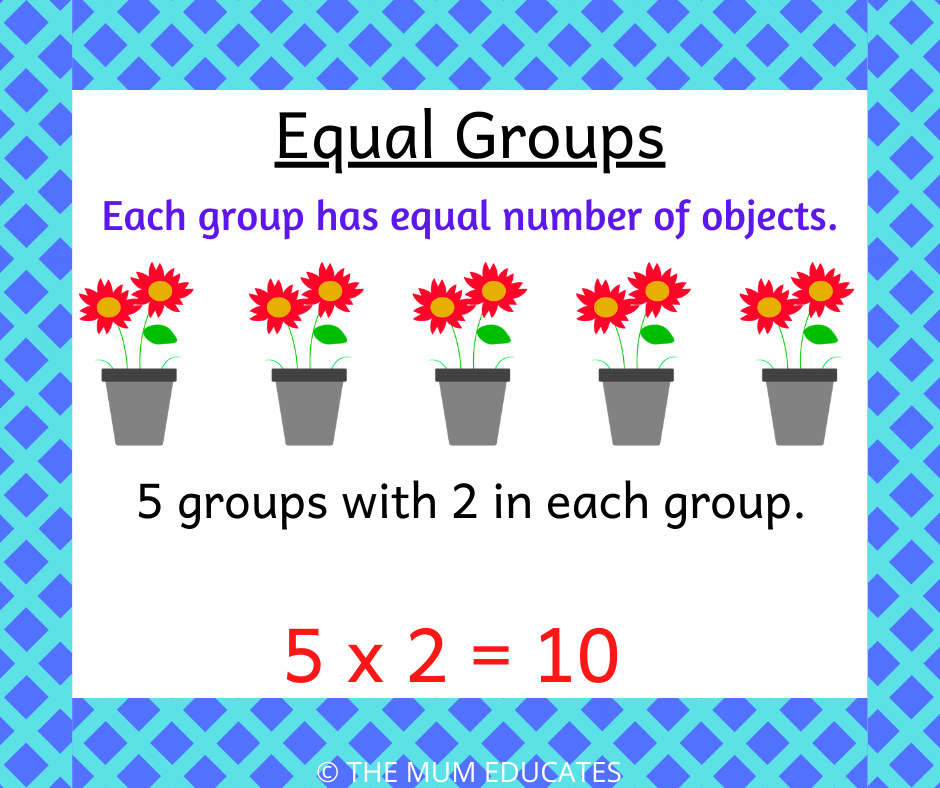3. Arrays:

So once children know repeated addition and equal groups, the next step is to introduce Arrays.  An array is an arrangement of objects in rows and columns. Each row holds the equal number of objects as the other rows in the array, as must the columns.

Example:

The example below shows 5 x 4=20.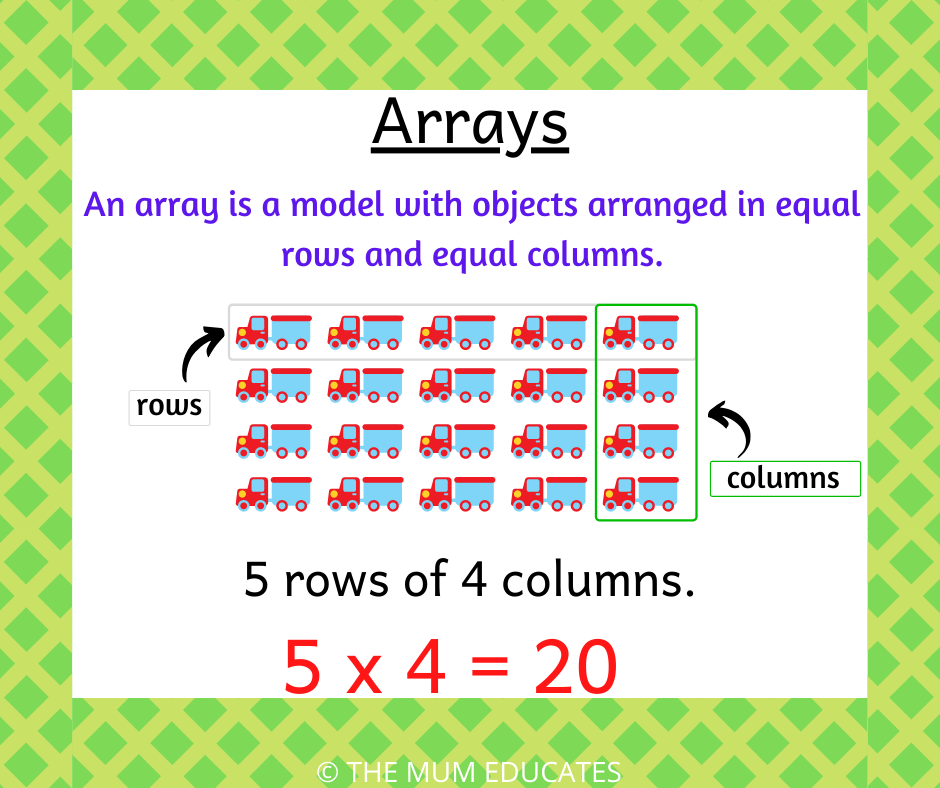4. Number Line:

Number lines are a fun way to teach multiplication. But to understand this concept, children must be fluent in counting ones forward and backwards. Once they are secure, they can skip count on the number line to find the answer.

Example:

Starting from zero, a frog hops 2cm four times.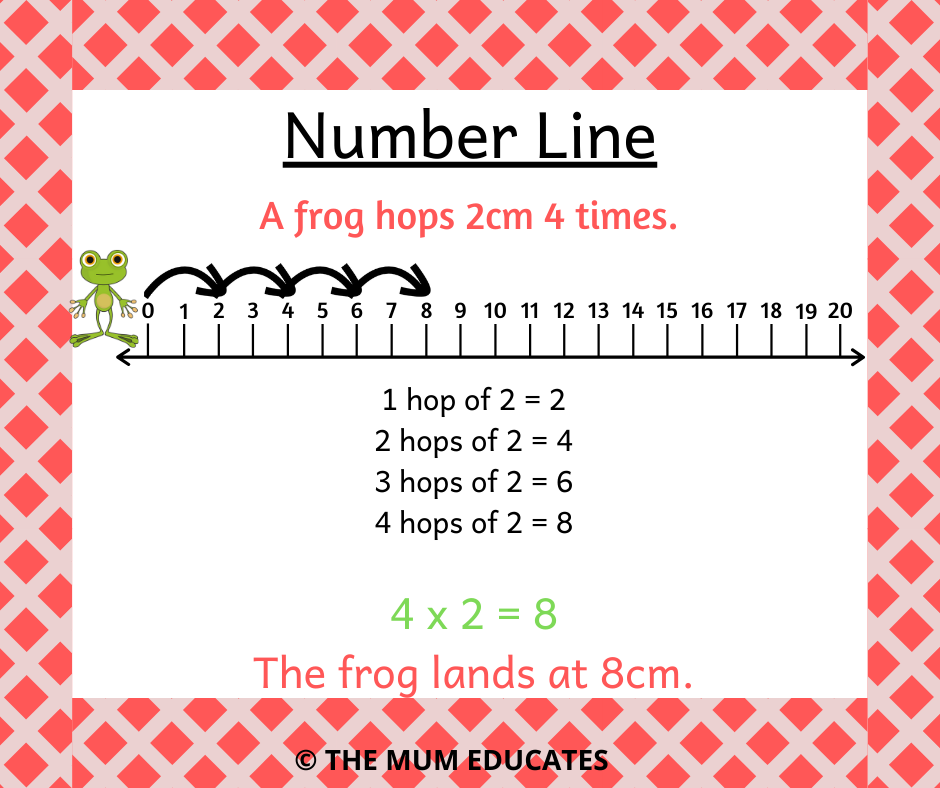#### Look out for real-life arrays:

Once the children learn all the strategies of multiplication ask them to find the real-life arrays around them.

Ask your children to write a multiplication sentence for the real-life pictures below.